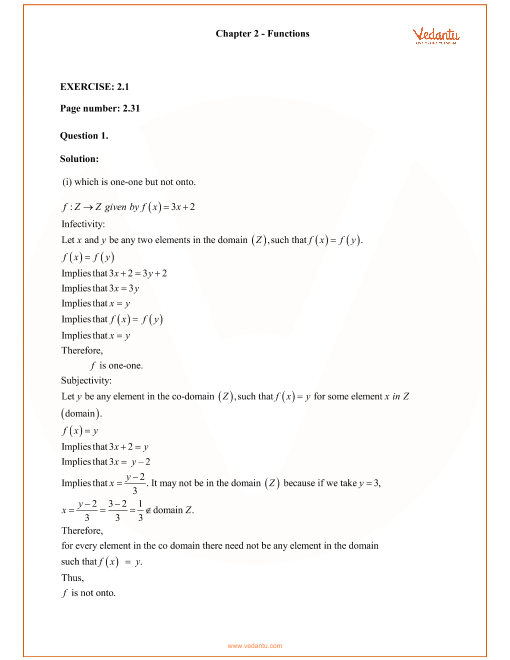RD Sharma Class 12 Maths Solutions Chapter 2 - Functions

RD Sharma Solutions for Class 12 Maths Chapter 2 - Functions - Free PDF Download

Functions are one of the most important chapters for Class 11 students. It is the basics of calculus that we study in higher classes. The free PDF of RD Sharma Class 12 Chapter 2 solutions are prepared by Vedantu experts to make the students understand easily. All solutions are particularly designed to provide students with a step-by-step approach so that they can prepare for their exams by referring to the solutions given.

Do you need help with your Homework? Are you preparing for Exams?
Study without Internet (Offline)Class 12 Maths RD Sharma Chapter 2 Solutions

We have provided step-by-step solutions for all exercise questions given in the pdf of Class 12 RD Sharma Chapter 2 - Functions. All the Exercise questions with solutions in Chapter 2 - Functions are given below:

Exercise 2.1

Exercise 2.2

Exercise 2.3

Functions are the relation between a set of inputs and a set of outputs with the property that each input map has exactly one output. The functions are generally called with a single letter, such as f. Functions may be thought of as a device in a box that is open at two ends. You place something in one end of the box, it somehow shifts inside the box, and then the result pops out of the other end. Some of the important concepts of Functions discussed in this chapter are as follows:

• Classification of Functions.

• Types of functions:

• Constant function

• Identity function

• Modulus function

• Integer function

• Exponential function

• Logarithmic function

• Reciprocal function

• Square root function

• Operations on real functions

• Kinds of functions

• One-one function

• On-to function

• Many one function

• In to function

• Bijection

• Properties of the composition of functions.

• Composition of functions.

• Composition of real function.

• Inverse of a function.

• Inverse of an element.

• Relation bw graphs of a function & its inverse.

Tips on How to Prepare for Exams Using Class 12 Maths RD Sharma Chapter 2 Solutions Free PDF

This RD Sharma Solutions Class 12 Maths Functions free PDF available on the Vedantu platform provides an excellent solution to all problems of the Textbook. Following tips will help students to ace their exams when preparing RD Sharma solutions of Functions.

• We advise students to read the questions carefully before attempting them. As the questions from Functions contain a few tricky questions, so if the questions are not understood properly, we may end up with the wrong answer.

• The PDF which is available for free on the Vedantu platform gives a step-by-step approach to a solution. So students are advised to have a glance through the content properly without missing any of the mentioned steps.

• The RD Sharma Solutions For Class 12 Maths Chapter 2 has lots of exercise problems and practice problems which helps students to practice before their exams.

Conclusion

After studying through Class 12 Maths RD Sharma Chapter 2 Solutions, students will be more confident when attempting any questions of Functions. RD Sharma's free PDF solutions are developed by talented experts to provide students with top-notch error-free solutions. Students can download a free copy of the PDF that is available on Vedantu's website.

1. Why Should I Refer Vedantu for RD Sharma Solutions for Class 12 Maths Chapter 2?

Ans: The solutions for RD Sharma Solutions For Class 12 Maths Chapter 2 are created by experts who have vast subject knowledge. A lot of research has been done to prepare strategies to offer step-by-step solutions for students to understand easily.

2. How Important are Class 12 Maths RD Sharma Chapter 2 Solutions?

Ans: From elementary calculus to multivariate calculus, and well beyond, the Functions has many significant applications. Also, Functions is one of the important topics in JEE exams. This chapter has a weightage of around 5-6% in both CBSE and JEE exams.

3. How do I get the Free RD Sharma Solutions Class 12 Maths Functions?

Ans: Students can download free PDF solutions of RD Sharma Solutions for Class 12 Maths - Functions on Vedantu’s platform which is developed according to NCERT curriculum.SHARETWEETSHARESUBSCRIBE